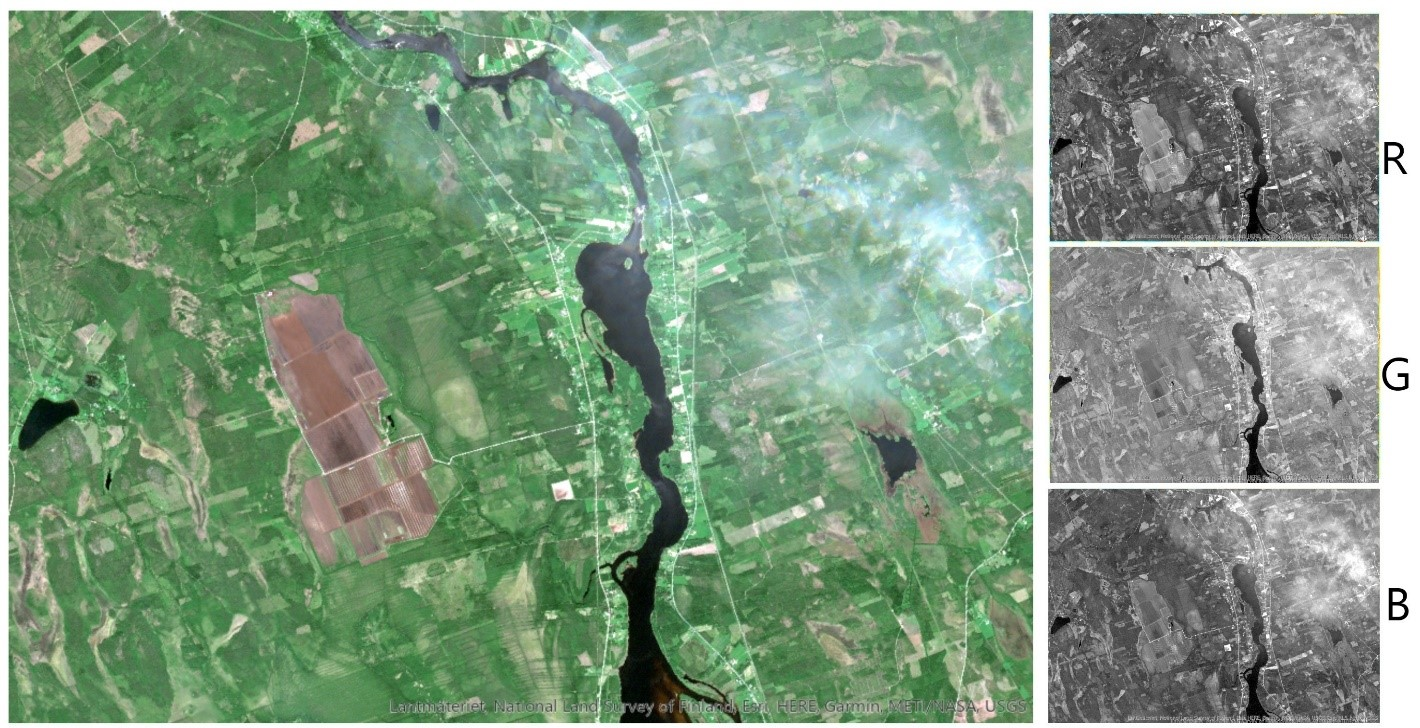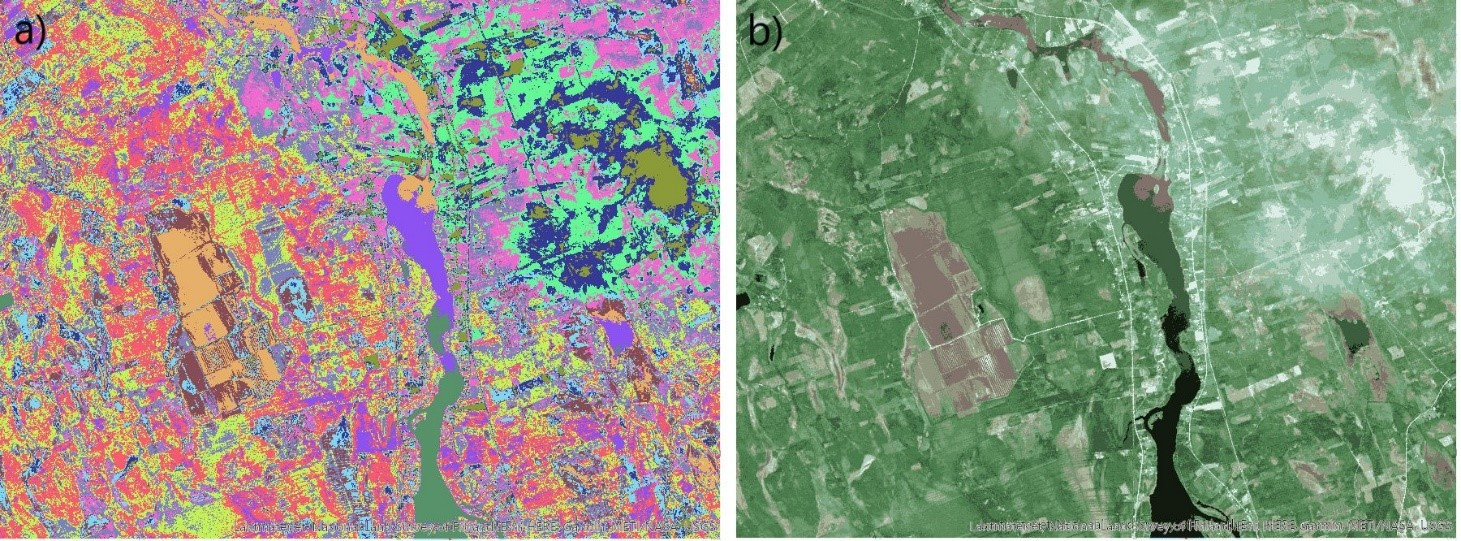•Stable Water ClusterForming a Double Helix

•Stable Water ClusterForming a Double Helix 1

What are multivariate datasets and self-organizing maps? And what do they have to do with geological data and machine learning? Johanna Torppa, the Senior Specialist at GTK, explains in a three-blog series the possibilities of GisSOM software in the visualization and analysis of geological datasets.
This blog was intended to present the GisSOM software, but its length exceeded the maximum feasible length of any kind of blog before the word GisSOM was even mentioned. The only solution was to divide the blog into a series of three blogs of which this is the first one. Here we, as the topic already reveals, describe multivariate data and multivariate clustering, and take a glance at what can be done with self-organizing maps.

## Multivariate Data

Consider, that we want to divide a satellite remote sensing RGB image (photograph) into subareas based on color. Our aim may be, for instance, to roughly separate water, clouds, forest, and fields from each other.Figure 1. Left: A satellite image (reflection of solar radiation in the red, green, and blue bands of the electromagnetic spectrum). Right: The red, green, and blue band reflectance represented as separate grayscale images. Our image (Figure 1) can be thought of comprising a set of data points (pixels) each having three variables representing reflected solar radiation in red, green, and blue bands of the electromagnetic spectrum. If you think this is obvious, you should be able to easily generalize this to having a larger number of variables for a pixel. Any dataset comprising more than one variable at each data point is called a multivariate dataset. In geoscientific research, the variables usually are something that we cannot see, like magnetic field intensity, electric conductivity, the concentration of different geochemical elements, pH, or whatever you may imagine. Any three of these variables could be visualized using the RGB color model – or even four variables by applying the transparency channel alpha. Most often, however, we have more than three or four variables, and direct visualization of all the variables using colors is not possible. The satellite image in Figure 1, with three variables, was chosen as one example dataset in this blog since the ability to visualize the variables helps to understand what is happening behind the scenes in the data analysis. All the methods discussed, however, can directly be applied to a dataset with any number of variables. Another reason to use a satellite image as an example is its spatial nature: data points with a spatial reference can be visualized as a map, giving data points a clear context and, again, facilitating understanding of what is happening to the data. We will, however, not be considering spatial data analysis methods. When dealing with spatial data, the spatial coordinates will be treated as passive parameters that are kept connected to each data point whatever happens to it, but they are not used in the data analysis.

## Multivariate Clustering

At the very beginning of this blog, we proposed an idea of dividing the satellite image to subregions based on color. For solving this problem, we have to dive into the field of clustering. A cluster is a group of similar data points and, contradictory to a class, does not have a predefined meaning or definition of representative variable values. A clustering scheme is specific to each individual dataset. This means, that if we take two random sub-samples of a dataset and cluster them separately using the same clustering method, we get two different clustering results. How different the results are, depends on the data: if the data contains clusters by nature, results are similar, while clustering sub-samples of a more or less uniformly distributed dataset are likely to produce totally different results. Real-life geoscientific datasets do not often contain clearly separable clusters. There are a large number of clustering techniques for multivariate data, some of which require a priori knowledge of the number of clusters, and others that find the optimal number in the clustering process. Even if this “optimal number of clusters” sounds promising, it does not mean “universally optimal”, but optimal using the selected method and process parameters. Actually, there rarely exists a single correct clustering scheme. In other words, there is no correct number of clusters or way to assign the data points in different clusters. Often one needs to run clustering several times, possibly using several clustering approaches, to map the possible solutions. Further analysis of the clustered data reveals how different clustering schemes serve in solving your research problem. As an example of a real-life clustering problem, let’s define subareas of differing colors in our satellite image (Figure 1). We apply ArcGIS’s Iso Clustering tool with 12 clusters.Figure 2. Clustering result for the satellite image using 12 clusters. Pixels colored a) using the clustered index, b) based on the red, green, and blue values representative of each cluster. In Figure 2, the clustered data points are mapped back to geospace. In addition to the R, G, and B values, the clustered data has a fourth variable, the clustered index, which is used to define the color of each pixel in Figure 2a. In Figure 2b, pixels are colored using the representative R, G, and B values for each variable in each cluster, obtained from the clustering process. Although the cluster colors represent the original data (Figure 1) fairly well based on visual inspection, this particular clustering result is not the only feasible one. We could have more or fewer clusters, and data points could be allocated to the clusters somewhat differently. To estimate the goodness of the clustering scheme, we can use a number of different metrics that measure the spread of the variable values within each cluster and the difference of the variable values between each pair of clusters. However, just like different clustering methods produce different cluster schemes, also different goodness of clustering metrics produce different results. Making the choice of which cluster scheme should be chosen as the final result or for further data analysis is by no means simple. One powerful method to help in obtaining a stable clustering result, and for visually representing the data and clusters even for a large number of variables, is the self-organizing maps method (SOM). SOM is effective also for simplifying a large data set or for predicting values for yet unobserved data.